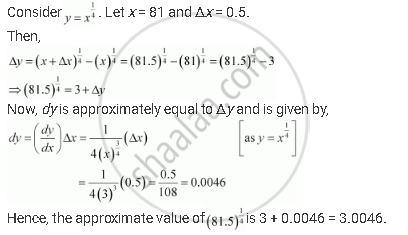Share

# Using Differentials, Find the Approximate Value of the Following up to 3 Places of Decimal (81.5)^(1/4) - CBSE (Commerce) Class 12 - Mathematics

#### Question

Using differentials, find the approximate value of the following up to 3 places of decimal

(81.5)^(1/4)

#### Solution

(81.5)^(1/4)Is there an error in this question or solution?

#### APPEARS IN

NCERT Solution for Mathematics Textbook for Class 12 (2018 to Current)
Chapter 6: Application of Derivatives
Q: 1.13 | Page no. 216

#### Video TutorialsVIEW ALL 

Solution Using Differentials, Find the Approximate Value of the Following up to 3 Places of Decimal (81.5)^(1/4) Concept: Approximations.
S